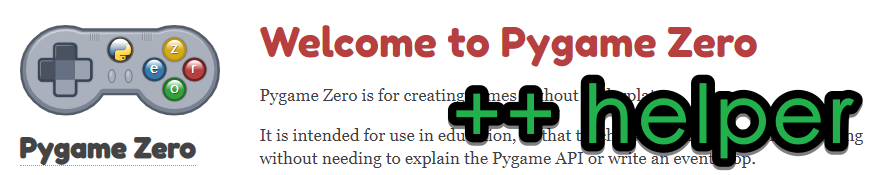# PygameZero助手（比照Scratch而做的增强）Pygame Zero少了一些Scratch中有的、游戏常用的函数。Pygame Zero Helper（PygameZero助手）就是为了弥补这一不足，提供了这些缺少的功能。

 ``` 1 2 3 4 5 6 7 8 9 10``` ``````import pgzrun from pgzhelper import * alien = Actor('alien') alien.flip_x = True def draw(): alien.draw() pgzrun.go() ``````

pgzhelper提供了如下这些函数：

## Actor.flip_x, Actor.flip_y#

 ```1 2``` ``````alien = Actor('alien') alien.flip_x = True ``````

## Actor.scale#

 ```1 2 3``` `````` alien = Actor('alien') alien.scale = 0.5 # 0.5倍大小 alien.scale = 2 # 2倍大小 ``````

## Actor.move_forward(), Actor.move_back(), Actor.move_right(), Actor.move_left()#

 ```1 2 3``` ``````alien = Actor('alien') alien.angle = 45 alien.move_forward(50) # Moves towards the North-East ``````

## Actor.direction, Actor.move_in_direction()#

 ```1 2 3``` ``````alien = Actor('alien') alien.direction = 45 # Does not rotate the actor alien.move_in_direction(50) # Moves towards the North-East ``````

## Actor.distance_to(), Actor.direction_to()#

 ```1 2 3 4``` ``````alien = Actor('alien') hero = Actor('hero') print(alien.distance_to(hero)) print(hero.direction_to(alien)) ``````

## Actor.move_towards(), Actor.point_towards()#

 ```1 2 3 4``` ``````alien = Actor('alien') hero = Actor('hero') hero.move_towards(alien, 5) alien.point_towards(hero) ``````

## Actor.get_rect()#

 ```1 2``` ``````alien = Actor('alien') screen.draw.rect(alien.get_rect(), (255,0,0)) # 相当于给alien画方框 ``````

## Actor.images, Actor.next_image()#

 ```1 2 3 4 5 6 7 8``` ``````alien = Actor('alien_run1') alien.images = ['alien_run1','alien_run2','alien_run3'] def update(): alien.next_image() def draw(): alien.draw() ``````

## Actor.fps, Actor.animate()#

 ```1 2 3 4 5 6 7 8 9``` `````` alien = Actor('alien_run1') alien.images = ['alien_run1','alien_run2','alien_run3'] alien.fps = 10 # 设置帧率 def update(): alien.animate() # 动画 def draw(): alien.draw() ``````

## Actor.collidepoint_pixel(), Actor.collide_pixel(), Actor.collidelist_pixel(), Actor.collidelistall_pixel()#

 ```1 2 3 4 5``` ``````alien = Actor('alien') def on_mouse_down(pos): if alien.collidepoint_pixel(pos): print("Eek!") ``````

## set_fullscreen(), set_windowed(), toggle_fullscreen(), hide_mouse(), show_mouse()#

 ```1 2 3 4 5``` ``````hide_mouse() def update(): if keyboard.f: toggle_fullscreen() ``````